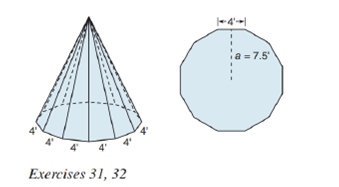Chapter 9.2, Problem 32EElementary Geometry For College St...

7th Edition
Alexander + 2 others
ISBN: 9781337614085

Solutions

Chapter
SectionElementary Geometry For College St...

7th Edition
Alexander + 2 others
ISBN: 9781337614085
Textbook Problem

For its occupants to be protected from the elements, it was necessary that the teepee in Exercise 31 be enclosed. Find the amount of area to be covered; that is, determine the lateral area of the rectangular dodecagonal pyramid. Recall that its altitude measures 15 f t .To determine

To find:

The lateral area of the pyramid.

Explanation

Given:

A teepee in shape of a regular pyramid with a dodecagon (12 sides) for the base as below,

and the height of the teepee is 15ft and side of the base as 4ft and apothem a=7.5ft.

Properties Used:

A pyramid is made by connecting a base to an apex. The base is flat with straight edges, no curves, hence, a polygon and all other faces are triangles.

A regular pyramid is a pyramid whose base is a regular polygon and whose lateral edges are all congruent.

The lateral area L of a regular pyramid with slant height of length l and perimeter P of the base is given by

L=12lP.

The total surface area T of a pyramid with lateral area L and base area B is given by

T=L+B.

According to the Pythagorean theorem, in a right-angled triangle

hypotenuse2=base2+perpendicular2.

In a regular pyramid, the lengths of the apothem a of the base, the altitude h, and the slant height l satisfy the Pythagorean Theorem; that is, l2=a2+h2

Still sussing out bartleby?

Check out a sample textbook solution.

See a sample solution

The Solution to Your Study Problems

Bartleby provides explanations to thousands of textbook problems written by our experts, many with advanced degrees!

Get Started